Default UI Menu: Constraints/SymmetricMakes two objects, or object endpoints, symmetric with respect to a symmetry axis.

1. Start with three lines - one of which is the symmetry line. In some cases the symmetry line will move as a result of this constraint, so if you want it to remain in place, fix it with a constraint such as Fix Geometry.

2. Activate the Symmetric constraint and select the first line.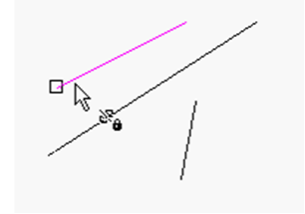1. Select the second line.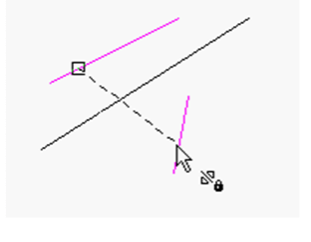1. Finally, select the symmetry line.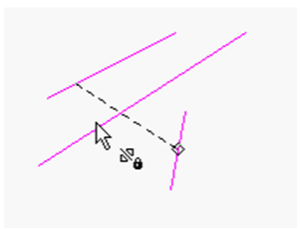The first two lines are now symmetric with respect to the third.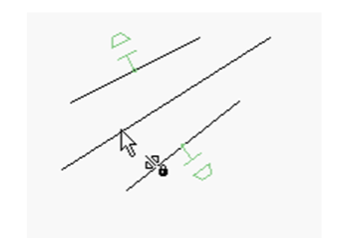1. You can also use this constraint on endpoints. Select Vertices 1 and 2, then select the symmetry line.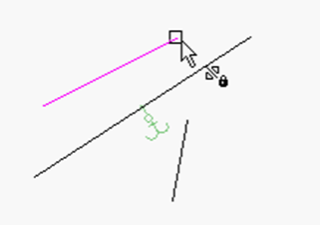The lines themselves keep their orientation, but their endpoints are symmetric.1. To make both lines completely symmetric, apply the Symmetric constraint to the other two endpoints.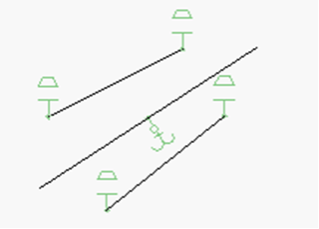1. This constraint can also be used on circles and arcs. If you select two arcs (not at their endpoints) . . .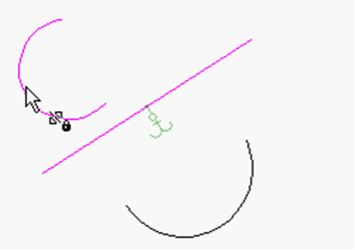... they will become symmetric and have equal radii.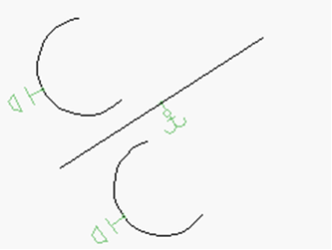1. Like with lines, you can apply the Symmetry constraint at both sets of endpoints. First the upper endpoints . . .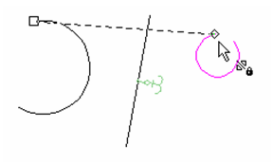1. . . . then the lower endpoints.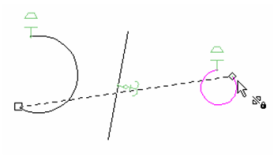The result is that both sets of endpoints are symmetric, but the orientations of the arcs are still different, because they have different radii.1. You can make the arcs completely symmetric by adding an Equal Radius constraint.Note: You could get the same results if you apply three Symmetric constraints - one to the arcs themselves, and one for both sets of endpoints.